##Projectivities play related to a triangle

This is a reworking of the ideas discussed in TriangleCircumconics.html . The image below displays the three projectivities related to a triangle and a distinguished point x in its plane. The image contains a fixed equilateral A0B0C0, its center M0, incircle i and circumcircle c. It contains also a triangle ABC its incenter I, centroid M and an arbitrary point x (not on the sides of ABC).
 Projectivity F (actual an affinity) maps A0 to A, B0 to B, C0 to C and M' to M. The in/circum-circle of A0B0C0 map correspondingly to the inner/outer Steiner ellipses e', e'' of ABC.
 Projectivity G fixes the vertices of the triangle and maps M to x. G maps the inner/outer Steiner ellipses to two conics e'(x), e''(x) correspondingly. G maps the line at infinity to the trilinear polar L(x) of x. Every line through x, intersects the conics at z1, z2 and L(x) at z3 so that z2, z3 are harmonic conjugate w.r. to z1, x: (x,z1,z2,z3)=-1. This relation generalizes the homothety relation between inner/outer Steiner ellipses.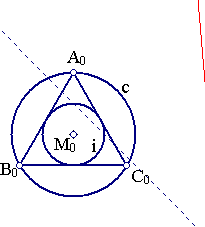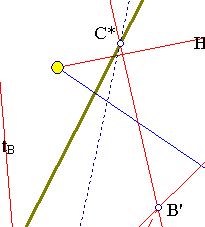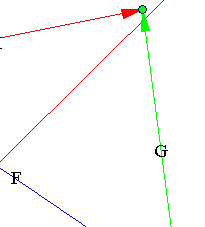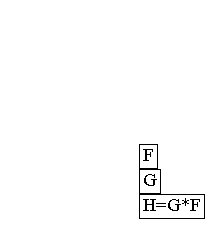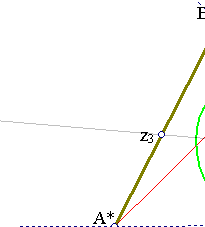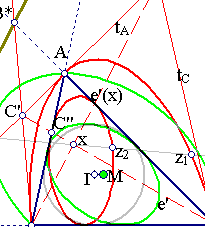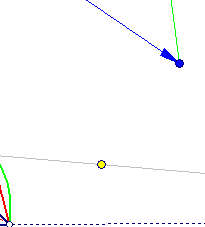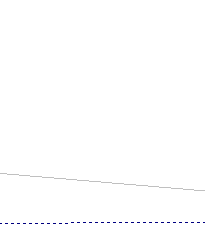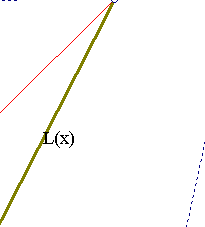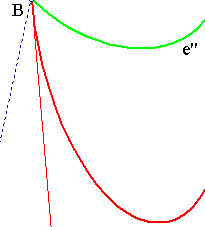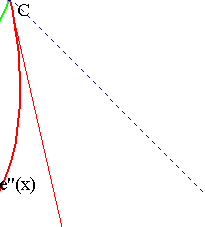Projectivity H can be defined directly by the requirement to map A0 to A, B0 to B, C0 to C and M0 to x. It is also the composition H = G*F. H maps the line at infinity to L(x). From the corresponding property of the equilateral follows that the line joining a vertex (f.e. C) with the intersection point of the tangents to e''(x) at the other vertices (f.e. C' intersection of tA, tB) passes through the contact point of e'(x) with the opposite side and passes also through x. Also the tangents of e''(x) at the vertices intersect the opposite sides at points A*, B*, C* lying on L(x).
 On tangent tA points A, A* are harmonic conjugate to C', B' and similar relations hold for cyclic permutations of the letters. Harmonic relations hold also for other quadruples of points f.e. (A,B,C'',C*)=-1, (C',x,C'',C)=-1
 Note that H maps the bundle of circles concentric to the circumcircle of the quadrilateral to the bundle of conics generated by the two conics e'(x) and e''(x). This is the same with the conics-bundle generated by one of these conics and L(x), considered as a double-line singular conic.
 Given the triangle ABC, the previous discussion shows that conics come always in pairs (e'(x), e''(x)) of in/circum-scribed. The pair is completely determined from the location of point x or the corresponding trilinear polar L(x). Given for example a circumscribing conic, one determines the line L(x) as the line containing the intersection points A*, B*, C* of the sides with the tangents at opposite vertices respectively. From L(x) one locates x and from this one proceeds to the construction of the corresponding inscribed conic.
 Each one of the conics e'(x), e''(x) is the dual of the other with respect to triangle ABC (see TriangleConics.html ). Given the triangle ABC, two arbitrary conics e', e'' one inscribed the other circumscribed in the triangle, in general, build not a pair of dual conics. For example the in/circum-circle of ABC are not in general dual of each other. The dual of the incircle is a particular conic and the dual of the circumcircle is another particular conic and not the incircle. These two special examples, corresponding to the duals of incircle and circumcircle are discussed in the references given below.
 By Poncelet's great theorem, taking a point A1 on the outer conic e''(x) and drawing the tangents to the inner e'(x) conic and their second intersection points B1, C1 with e''(x) we determine a triangle A1B1C1, which is also circumscribed in e'(x). This subject is discussed in ProjectiveRotations.html .

CircumcircleConjugate.html
CrossRatio.html
CrossRatio0.html
CrossRatioLines.html
GoodParametrization.html
Harmonic.html
Harmonic_Bundle.html
HomographicRelation.html
HomographicRelationExample.html
IncircleConjugate.html
Pascal.html
PascalOnTriangles.html
ProjectivityFixingVertices.html
ProjectiveRotations.html
Steiner_Ellipse.html
TriangleCircumconics.html
TriangleConics.html
TriangleCircumconics2.html
TrilinearPolar.html
 Produced with EucliDraw© http://users.math.uoc.gr/~pamfilos/